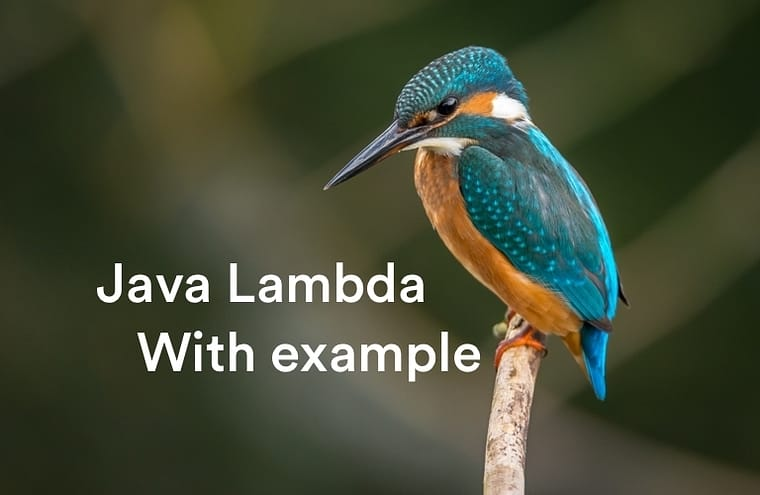# KieBlog# Java Lambda with example – You’re Happy and You Know It!

## 1 What’s Java Lambda

Let begin with definition about Java Lambda. What’s Java Lambda?

Lambda expressions basically express instances of functional interfaces (An interface with single abstract method is called functional interface.

Before you want to understand “What’s Lambda?”, you should understand functional interfaces first.

Oh ho bro, what’s functional interface?.

The functional interfaces is the interface with single abstract method is called functional interface.

Please remember that any interface contain only abstract method is functional interface

I understand the definition. But can you show me the syntax to use Java Lambda?. OK, let me show you a syntax.

LAMBDA SYNTAX = lambda operator -> body

If we use lambda with List, the lambda operator is each item in list. The body is what you want to do with each item. Imagination, it’s easy to understand with arrow (->).

The Java Lambda always use with arrow, which mean item -> what you want to do with item. It’s easy to understand.

• A function that can be created without belonging to any class. That’s a new thing only exist in Java 8 and many version in the future. With Java lambda, now we can call a function (in case they don’t belong to any class)
• A lambda expression can be passed around as if it was an object and executed on demand. (We can pass a function to call in manytimes with lambda)
• Of course, write short code and easy to understand. From Java 8, with Stream, Predicate and Lambda, we write less code and it’s look beautiful

### 1.2 Parameter

Lambda accept many way, many type to pass parameter. You can pass zero, one, two, multi parameter to Lambda if you need it.

#### 1.2.1 Zero parameter

We can write lambda with zero parameter in case the body no need any parameter.

`() -> System.out.println("This is KieBlog");`

#### 1.2.2 One Parameter

If method in body need one paramater, just do:

`parameter1 -> System.out.println("It's mean they need one: " + parameter1);`

#### 1.2.3 More than two parameter

If body need more than two parameter, just bring all of it to parentheses

```(parameter1, parameter2) ->
System.out.println("Need two or more: " + parameter1 + ", " + parameter2);```

## 3. Java lambda example

### 3.1 First easy example

```// This example very is to understand
public static void main(String args[])
{
// You have one list -> print each item in list
ArrayList<String> KieBlogArray = new ArrayList<String>();
}```

Use Java lambda to println each item in KieblogArray, we can do:

```// This example very is to understand
public static void main(String args[])
{
// You have one list -> print each item in list
ArrayList<String> KieBlogArray = new ArrayList<String>();
// This is lambda (print each n in KieBlogArray
KieBlogArray.forEach(n -> System.out.println(n));
}```

### 3.2 Execute function in body

Please take a look back to syntax, we have a body

```// If you want to do a sample expression in body
// Just do it in body
public static void main(String args[])
{
(str1, str2) -> {
System.out.println("String after concat:" + str1 + str2);
}
}```

### 3.3 Lambda with many condition in body

With lambda, refactor code is very easy, please remove complex thing to check the condition in your mind. Now, we can do it like:

```// We can use Lambda to handle complex condition
public static void main(String args[])
{
// With each item, it's easy to handle many condition
p -> p.getCity() == Person.City.CURRENT_CITY
&& p.getAge() >= 18
&& p.getAge() <= 25
}```

### 3.4 Define a function and call it

Use lambda, we can call a static function in another class with ::

```// Create a static function and call it with lambda
public class KieBlogClass{
public static boolean isDivideByTwo(int number1){
return (number1 % 2 == 0) ? true : false;
}
}

// Create a interface
public interface Divide {
public boolean divide(int number 1);
}

// We can call this function with lambda like this
Divide isDivideByTwo = MyClass::isDivideByTwo;```

### 3.5 Return what you want in Lambda

If you want to check any thing, or return any thing after check. Just write a lambda and return it. Like this

```// With one parameter
public static void main(String args[])
{
// Check if the number can divide by 2
(number) -> {
if (number % 2 == 0) {
return true;
}
return false;
}
}```
```// With two parameter
public static void main(String args[])
{
// If both can divide by 2
(number1, number2) -> {
if (number % 2 == 0 && number2 % 2 == 0) {
return true;
}
return false;
}
}```

### 3.6 Use Java Lambda with Comparator

Fitstly, define a Comparator like this:

```// Lambda with comparator
public interface KieBlogComparator {
// Check if length of str1 is longer than str2
public boolean compare(String str1, String str2);
}```

Now, we need to define what we do with comparator

```public interface KieBlogComparator {
KieBlogComparator kieComparator = (str1, str2)
-> return str1.length() > str2.length();
// Now use it
boolean str1LongerThanStr2 = kieComparator.compare("KieBlog", "Kie");
}```

### 3.7 Lambda with method reference

From Java 8, we can use Method Reference with syntax

SYNTAX: Object :: methodName

```// Use lambda like method reference
public static void main(String args[])
{
// Do it with lambda
KieBlog<String> c = s -> System.out.println(s);
// Or with method reference
KieBlog<String> c = System.out::println;
// What's easy to write?
}```

## 4. Reference